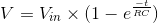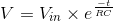# RC Time Constant

## Working principle

This model shows how a capacitor charges with a DC source and then discharges through a resistor. The table below the waveforms displays the time constant of the capacitor (the RC value), the peak voltage reached during the simulation and the voltage reached after one time constant (or at 63.2% of the capacitor’s total capacity). The user may define the time of the step, which disconnects the source from the capacitor and initiates the discharging phase.

### Useful formulas

Charging phase:Discharging phase:### Experiment

• Change the time constant by either increasing or reducing the RC value and observe the amount of time it takes for the capacitor to charge and discharge.
• You may notice that a resistance value of 0 Ohms is not possible here. Why is this?